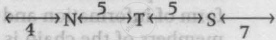# N Ranks Fifth in a Class. S is Eighth from the Last. If T is Sixth After N and is in the Middle of N and S, Then How Many Students Are There in the Class? - Logical Reasoning

MCQ

N ranks fifth in a class. S is eighth from the last. If T is sixth after N and is in the middle of N and S, then how many students are there in the class?

• 23

• 24

• 25

• 26

#### Solution

24
Explanation:∴ Number of students in the class
= (4 + 1 + 5 + 1 + 5 + 1 + 7) = 24

Concept: Ranking (Entrance Exam)
Is there an error in this question or solution?